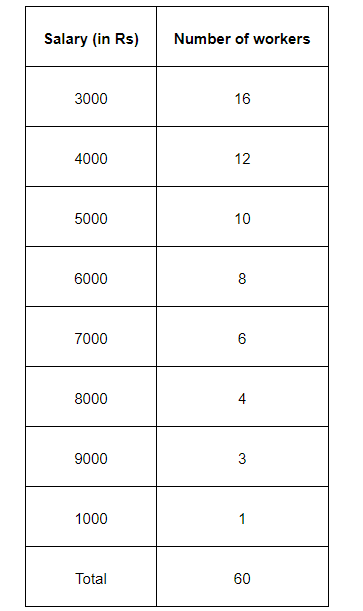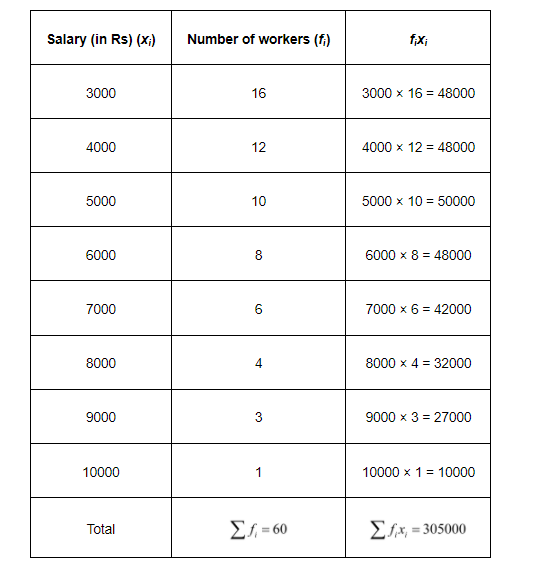# Find the mean salary of 60 workers of a factory from the following table:

Question.

Find the mean salary of 60 workers of a factory from the following table:Solution:

We know that

Mean $=\frac{\sum f_{i} x_{i}}{\sum f_{i}}$

The value of $\sum f_{i} x_{i}$ and $\sum f_{i}$ can be calculated as follows.Mean salary $=\frac{305000}{60}$

$=5083.33$

Therefore, mean salary of 60 workers is Rs 5083.33.